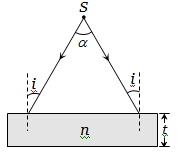NEET Physics Ray Optics and Optical Instruments Questions Solved

A diverging beam of light from a point source S having divergence angle $\alpha$, falls symmetrically on a glass slab as shown. The angles of incidence of the two extreme rays are equal. If the thickness of the glass slab is t and the refractive index n, then the divergence angle of the emergent beam is(a) Zero                                       (b) $\alpha$

(c) ${\mathrm{sin}}^{-1}\left(1/n\right)$                           (d) $2{\mathrm{sin}}^{-1}\left(1/n\right)$

Explanation is a part of a Paid Course. To view Explanation Please buy the course.

Difficulty Level: